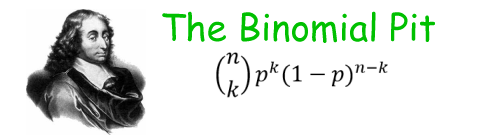### Binomial Probability Calculator

Enter n and p above, or pass them as a querystring. Example: http://anesi.com/binomial.htm?p=.5&n=10, the classic example of tossing a fair coin ten times. Or better, tossing 1,000 times.

Note: Numbers displayed in the results table and chart tooltips show 10 significant digits and scale from about 10-322 to 10322. Cumulative probabilities approach 1; if the significand rounds to 1, they just show 1. Display ends when k=n, or when one or more factors go out of range. If a factor goes out of range, a message is displayed showing the factor and its JavaScript value. When that occurs, note that a JavaScript value of zero or "infinity" does not really mean zero or infinity, just a positive number smaller or larger than the range of JavaScript's floating point representation. When the calculator is run, the URL in the address bar is updated with a querystring matching the arguments provided. Charting is done with the Highcharts JavaScript library, which we highly recommend.

Example: At the Monte Carlo casino on August 18, 1913, the roulette wheel came up black 26 times in a row. It was a single zero wheel, so the probability of black on each spin was 18/37 = ~.486486486. What was the probability of 26 black in a row? . (This is actually a bad example since you could just compute (18/37)26 and get the answer, but what the heck.)

Example for my grandkids: Using this calculator to solve the old "birthday problem" -- given a group of n people, what are the chances that two or more people have the same birthday? -- requires a two-step approach. Say the group size is 23. First thing is to find how many distinct pairs of people you can make from a group of 23, that is, the binomial coefficient 23 choose two. So run the calculator with 23 in the n box and anything (say .5) in the p box. The binomial coefficient shown in the full data table for n=23 and k=2 is 253. So you have 253 possible pairs of people. Now, run the calculator with 253 as n, and 1/365 (0.00273973) as p (we're disregarding leap years). You have a ~50% (p=0.4995223425) chance of no pairs with a shared birthday, and thus a ~50% chance of one or more pairs each with a shared birthday. If you take the group size to 70, 70 choose 2 is 2415, and the chance of no pair with a shared birthday falls to 0.13%. Of course, if you had a group of 366 people (disregarding leap years, or 367 if you include leap years), obviously the probability of no shared birthdays would be zero, not some very tiny positive but finite number, as the binomial distribution predicts. But since 366 choose 2 is 66795, the probability of no shared birthdays is 2.599704056 x 10-80, which is about equal to one, divided by the number of atoms in the observable universe, so it's close enough to zero not to worry about.